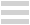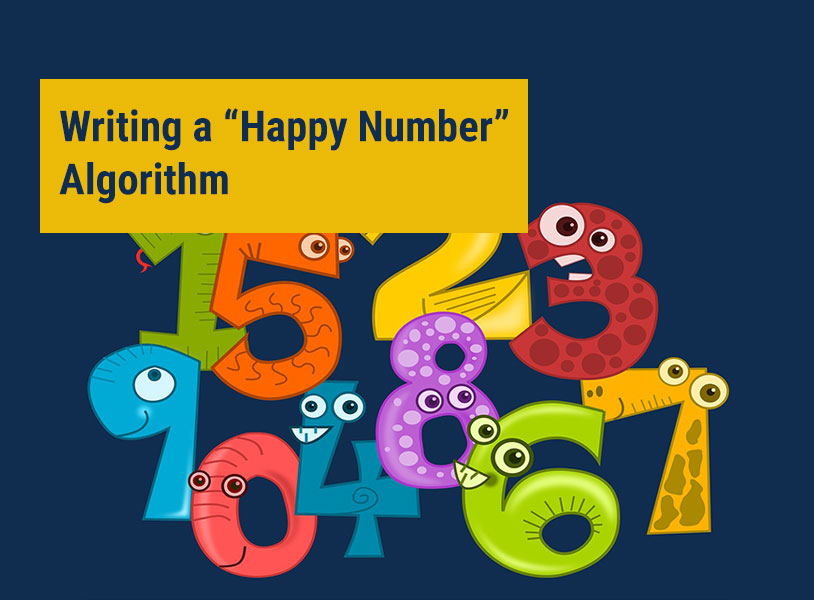# Writing a “Happy Number” AlgorithmAlgorithms play a huge role in computer programming. An algorithm isn't directly executed in programming but the ideas in the same algorithm act as the foundation of a code, thereby making it easier for the programmer to write a flawless and effective code. To write a code flawlessly, you should learn to write an algorithm first. It's basically the step-wise solution of a problem written in a story-telling fashion without involving all the technicalities of a specific programming language. An algorithm therefore is universal and the same ideas can be brought to life in any programming language. Today, we'll see the basic ways to write a “Happy Number” algorithm.

## Happy Number: A Basic Idea

How can numbers have emotions? They are abstract in nature. Hence, we can safely say that finding out numbers that are “happy” doesn't make the numbers happy. It makes the finder “happy”. A number can be deemed a “happy number” if it satisfies the following process or calculations performed on the same:

There's a condition at first to find out whether a number is “happy” or not. The given number must be a positive integer. The second step is to find the square of every digit present in the number. The third step is to find the sum of the individual squares of the digits found in the previous step. If the final answer after third step is found to be “1”, stop any further calculation. Otherwise, it's necessary to repeat this entire process cumulatively until the result becomes “1”. If it's possible to find the result “1” in the end, the given number is a “happy number”. If after repeated calculations it's determined that it's impossible to get the final result as “1”, the given number is “unhappy”.

Examples

Let's go through some examples to understand this concept easily.

Given number: 19.

The given number is a positive integer. Hence, it's possible to continue with the required calculations. Determine the square of each digit and find the sum.

1^2 + 9^2 = 1*1 + 9*9 = 82

The answer is not the desired “1”. So that means the process needs to be repeated.

8^2 + 2^2 = 68

6^2 + 8^2 = 100

1^2 + 0 + 0 = 1

Finally, we get our desired result “1”. Hence, the given number (19) is a happy number.

Given number =2.

It's a positive integer and hence, let's go through with the calculations.

2^2 = 4...................(i)

4^2= 16

1^2 + 6^2 = 37

3^2 + 7^2 = 58.

5^2 + 8^2= 89

8^2 + 9^2= 145

1^2 + 4^2+ 5^2= 42

4^2 + 2^2= 20

2^2 + 0 = 4............(ii)

Let's stop at this point. Did you find a pattern? You see that the last step (ii) has got a result of a number (4) that was already found previously (i). So that means it's virtually an endless loop and the answer will never be “1” in this case. Try to find such patterns in your calculations of other problems to save effort and time. Hence, the given number “2” is an unhappy number. There's a thumb rule for this process which can be consulted also. If the result at any stage of the calculation is a number that lies among the list of 4, 16, 20, 37, 42, 58, 89, 145, the given number in the problem is surely an unhappy number.

Another point to remember is that a happy number is not affected by rearranging the constituent digits. It means that 91 is also a happy number since we have already found out previously that 19 is a happy number. The happy number is also unaffected if a 0 is inserted anywhere or removed from somewhere. As per the two rules mentioned above, the numbers 19, 190, 109, 901 are all happy numbers.

## Algorithm

Before we start with the algorithm it's necessary to understand the conditional statements first because such statements are implemented in this algorithm in the form of a statement and in the code that would be based on the algorithm with all other technicalities. We'll deal with only the algorithm in this article. The conditional statement depends on more than one possible condition occurrence according to the problem. Like if “A” happens, “B” will be executed otherwise “C” will be executed. Let's get to the algorithm of finding out whether a given number is “happy” or not.

1. Start.
2. Accept the “user input number”.
3. If the “user input number” is a negative integer,

Display “Please insert a positive integer”.

else

continue to step 4.

4. Extract the digits from the user input number.

5. Square each digit individually and add them up to get a single final result which is stored in a variable (say, Z).

6. User input number = Z

7. If user input number is present in the list of 4, 16, 20, 37, 42, 58, 89, 145

Display “The number is :(”

else,

If user input number is “1”

Display “The number is :)”

else,

Repeat step 4.

8. Stop.

These types of basic problems are pretty interesting from the point of view of beginner's coding and mathematics for developing a clear concept of the subjects. Advanced mathematics and coding after-school classes can help you out immensely if you look to adopt an effective STEM education which can be deemed a part of the basic literacy in the modern world. These classes are immensely fun as well. We hope that this article based on “happy numbers” has made you happy as well. So keep on practising algorithms with a smile on your face and you'll be benefited for sure.### FUTURITE

Futurite aims to bring high quality STEM (Science, Technology, Engineering and Mathematics) education to school students in India. Our mission is to use cutting-edge technologies such as Robotics and 3D Printing to impart a thorough grounding in STEM subjects and concepts to school students while making their learning experience fun, engaging and hands-on. Futurite has been operating in Dubai successfully (as Premier Genie) since a number of years, working with over 25 of the top international schools and teaching 1000+ students. Futurite has been founded by alumni from IIT, IIM, JU and HEC Paris.